Courses

# Normal Shocks (Part - 1) Civil Engineering (CE) Notes | EduRev

## Civil Engineering (CE) : Normal Shocks (Part - 1) Civil Engineering (CE) Notes | EduRev

The document Normal Shocks (Part - 1) Civil Engineering (CE) Notes | EduRev is a part of the Civil Engineering (CE) Course Fluid Mechanics - Notes, Videos, MCQs & PPTs.
All you need of Civil Engineering (CE) at this link: Civil Engineering (CE)

Normal Shocks

• Shock waves are highly localized irreversibilities in the flow .

• Within the distance of a mean free path, the flow passes from a supersonic to a subsonic state, the velocity decreases suddenly and the pressure rises sharply. A shock is said to have occurred if there is an abrupt reduction of velocity in the downstream in course of a supersonic flow in a passage or around a body.

• Normal shocks are substantially perpendicular to the flow and oblique shocks are inclined at any angle

• Shock formation is possible for confined flows as well as for external flows.

• Normal shock and oblique shock may mutually interact to make another shock pattern.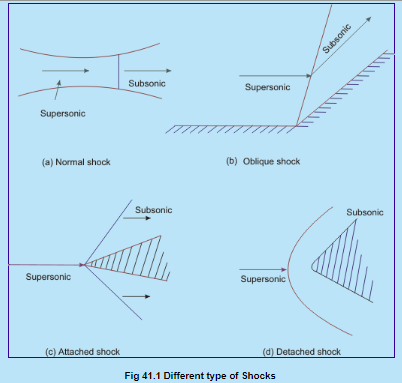Figure below shows a control surface that includes a normal shock.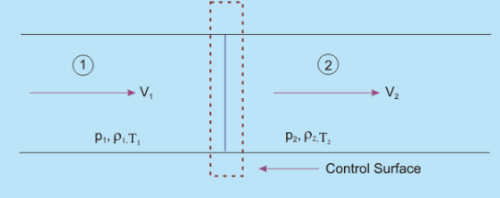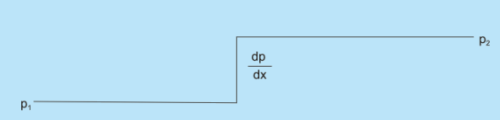Fig 41.2 One Dimensional Normal Shock

• The fluid is assumed to be in thermodynamic equilibrium upstream and downstream of the shock, the properties of which are designated by the subscripts 1 and 2, respectively. (Fig 41.2).

Continuity equation can be written as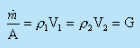(41.1)

where G is the mass velocity kg/ m2 s, and

From momentum equation, we can write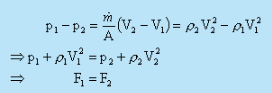(41.2b)

where p + ρV2 is termed as Impulse Function .

The energy equation is written as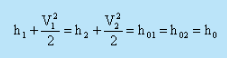(41.3)

where his stagnation enthalpy.

From the second law of thermodynamics, we know

: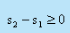To calculate the entropy change, we have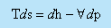For an ideal gas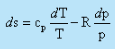For an ideal gas the equation of state can be written as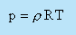(41.4)

For constant specific heat, the above equation can be integrated to give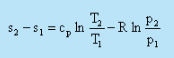(41.5)

Equations (41.1), (41.2a), (41.3), (41.4) and (41.5) are the governing equations for the flow of an ideal gas through normal shock.

If all the properties at state 1 (upstream of the shock) are known, then we have six unknowns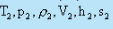in these five equations.

We know relationship between h and T [Eq. (38.17)] for an ideal gas,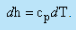For an ideal gas with constant specific heats,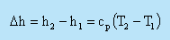Thus, we have the situation of six equations and six unknowns.

• If all the conditions at state "1"(immediately upstream of the shock) are known, how many possible states 2 (immediate downstream of the shock) are there? The mathematical answer indicates that there is a unique state 2 for a given state 1.

Fanno Line Flows

• If we consider a problem of frictional adiabatic flow through a duct, the governing Eqs (41.1), (41.3), (38.8), (41.5) and (41.6) are valid between any two points "1" and "2".

• Equation (41.2a) requires to be modified in order to take into account the frictional force, Rx, of the duct wall on the flow and we obtain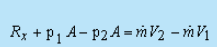So, for a frictional flow, we have the situation of six equations and seven unknowns.

• If all the conditions of "1" are known, the no. of possible states for "2" is 2. With an infinite number of possible states "2" for a given state "1", what do we observe if all possible states "2" are plotted on a T - s diagram, The locus of all possible states "2" reachable from state "1" is a continuous curve passing through state "1". The question is how to determine this curve? The simplest way is to assume different values of T2. For an assumed value of T2, the corresponding values of all other properties at " 2 " and Rx can be determined.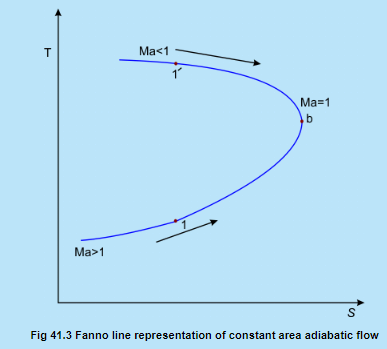• The locus of all possible downstream states is called Fanno line and is shown in Fig. 41.3. Point " b " corresponds to maximum entropy where the flow is sonic. This point splits the Fanno line into subsonic (upper)and supersonic (lower) portions.

• If the inlet flow is supersonic and corresponds to point 1 in Fig. 41.3, then friction causes the downstream flow to move closer to point "b" with a consequent decrease of Mach number towards unity.

• Note that each point on the curve between point 1 and "b" corresponds to a certain duct length L. As L is made larger, the conditions at the exit move closer to point "b". Finally, for a certain value of L, the flow becomes sonic.
Any further increase in L is not possible without a drastic revision of the inlet conditions.

• Consider the alternative case where the inlet flow is subsonic, say, given the point 1' in Fig. 41.3. As L increases, the exit conditions move closer to point "b". If L is increased to a sufficiently large value, then point "b" is reached and the flow at the exit becomes sonic. The flow is again choked and any further increase in L is not possible without an adjustment of the inlet conditions.

Offer running on EduRev: Apply code STAYHOME200 to get INR 200 off on our premium plan EduRev Infinity!

,

,

,

,

,

,

,

,

,

,

,

,

,

,

,

,

,

,

,

,

,

;